### Select a collection below for the respective carton calculator.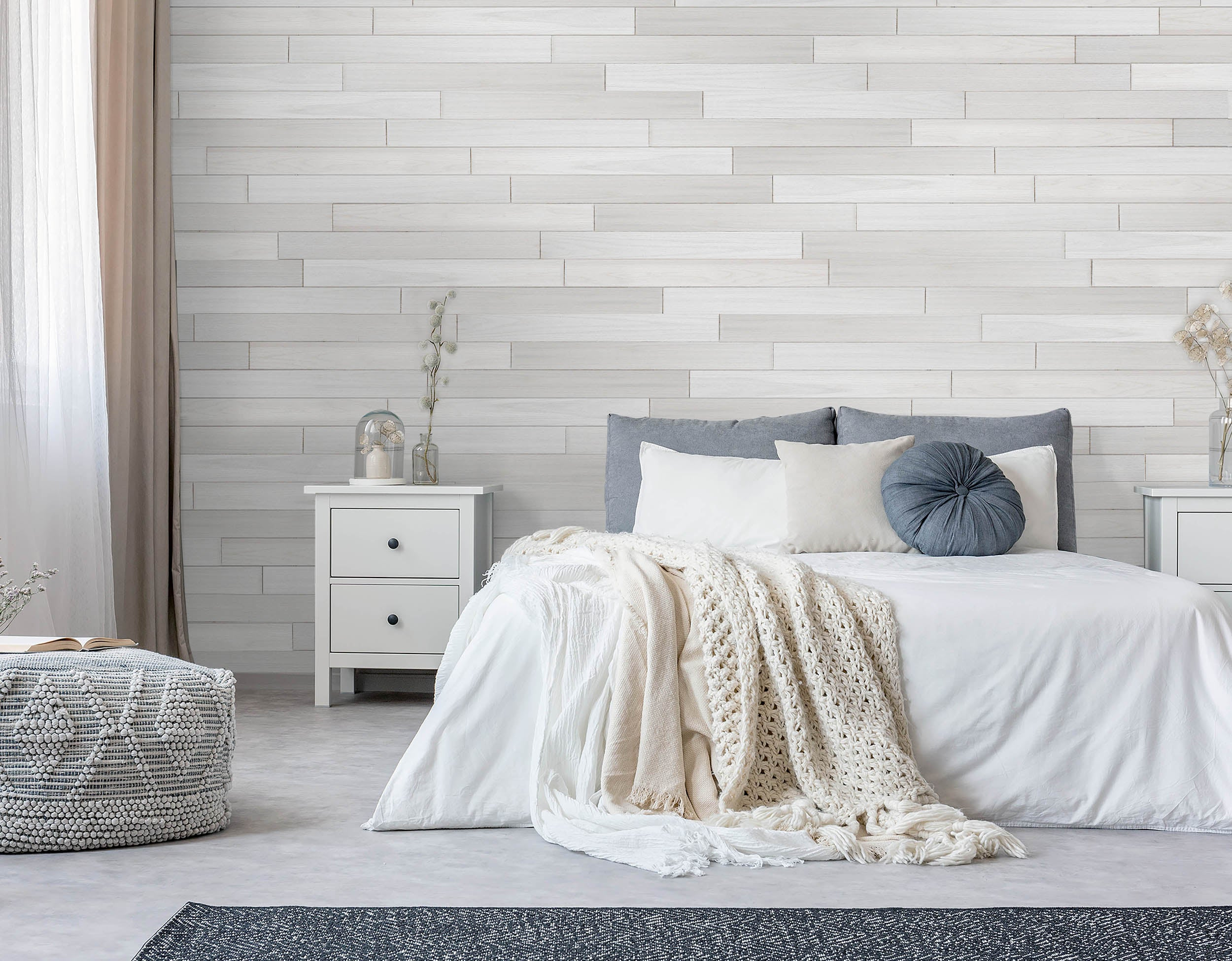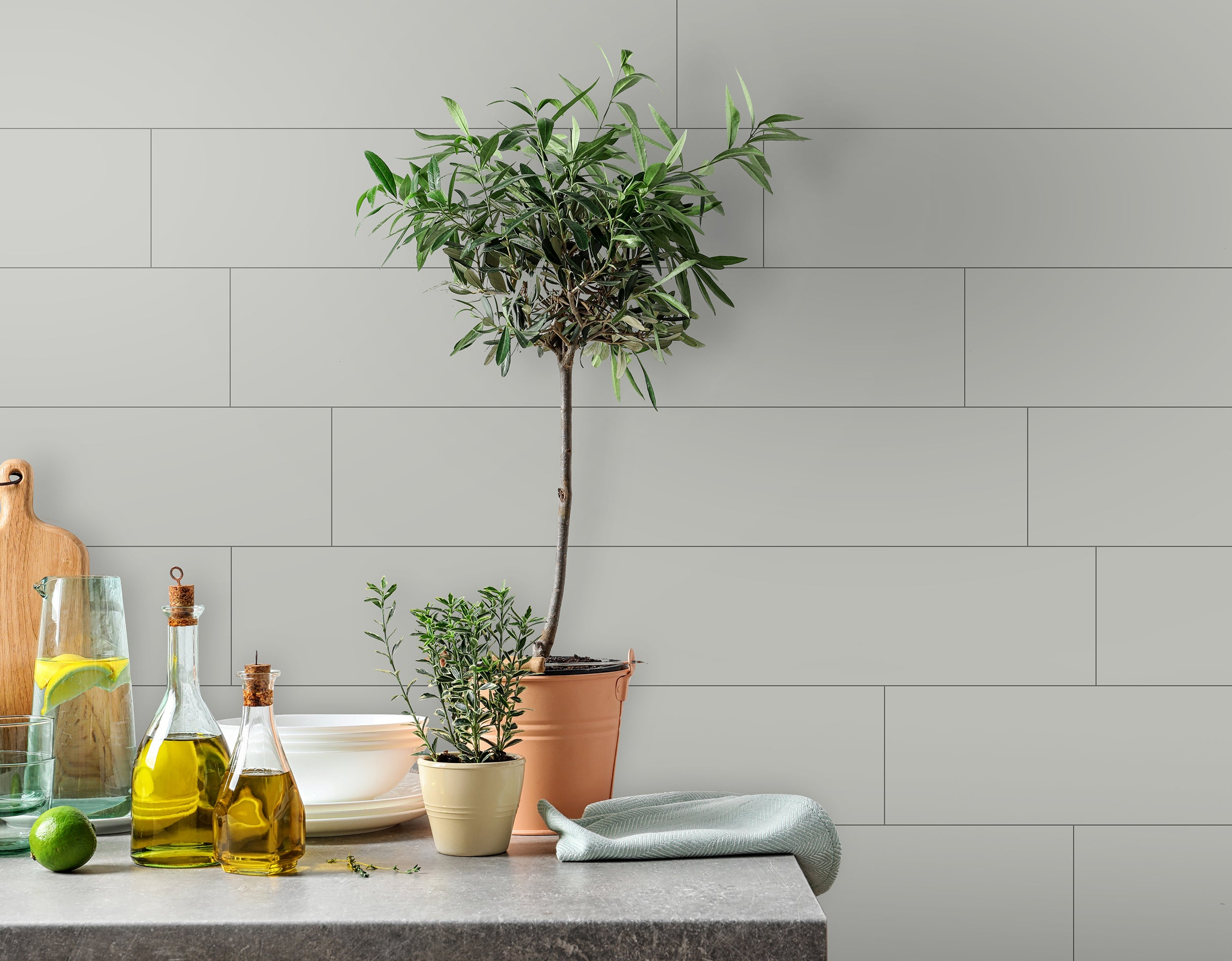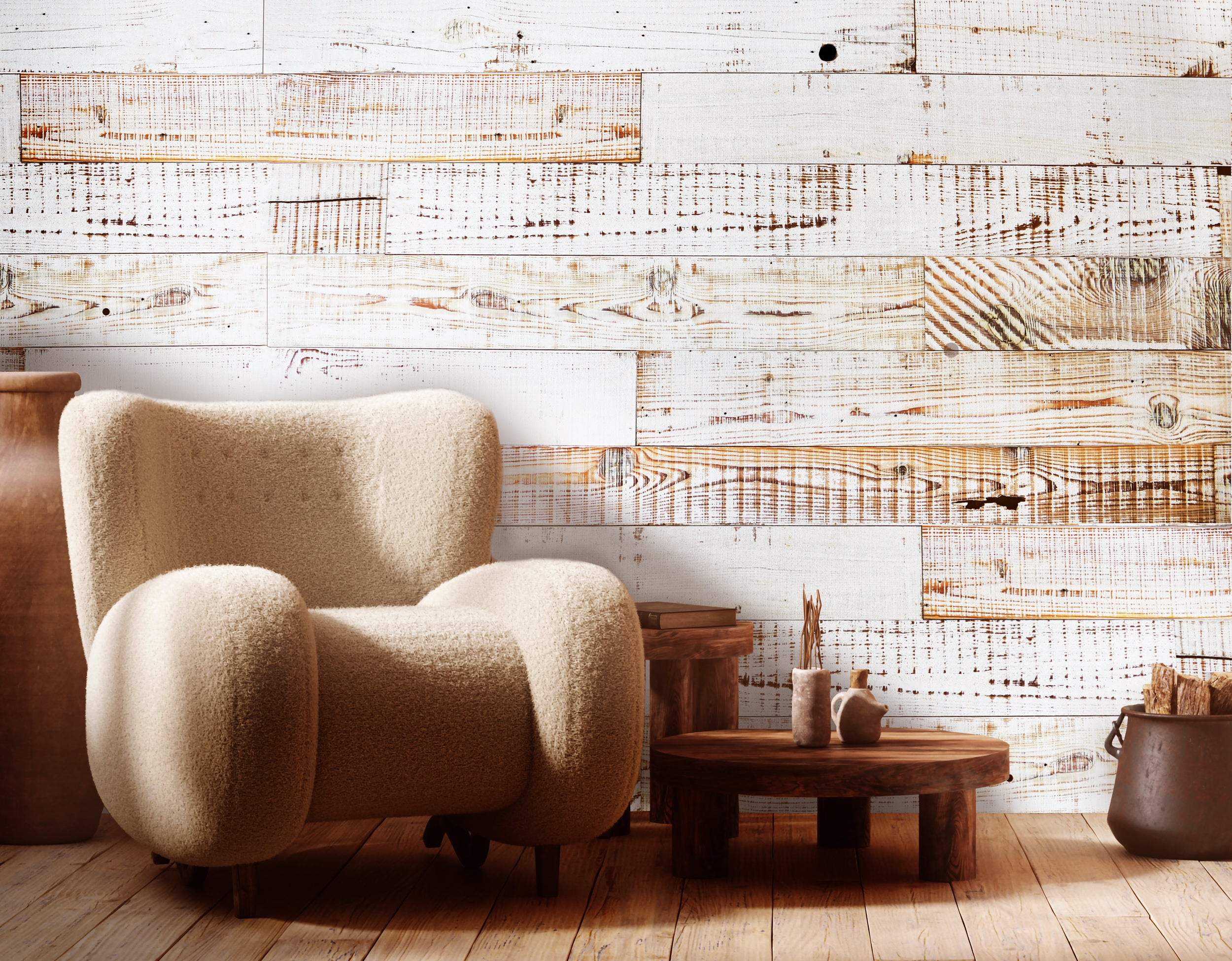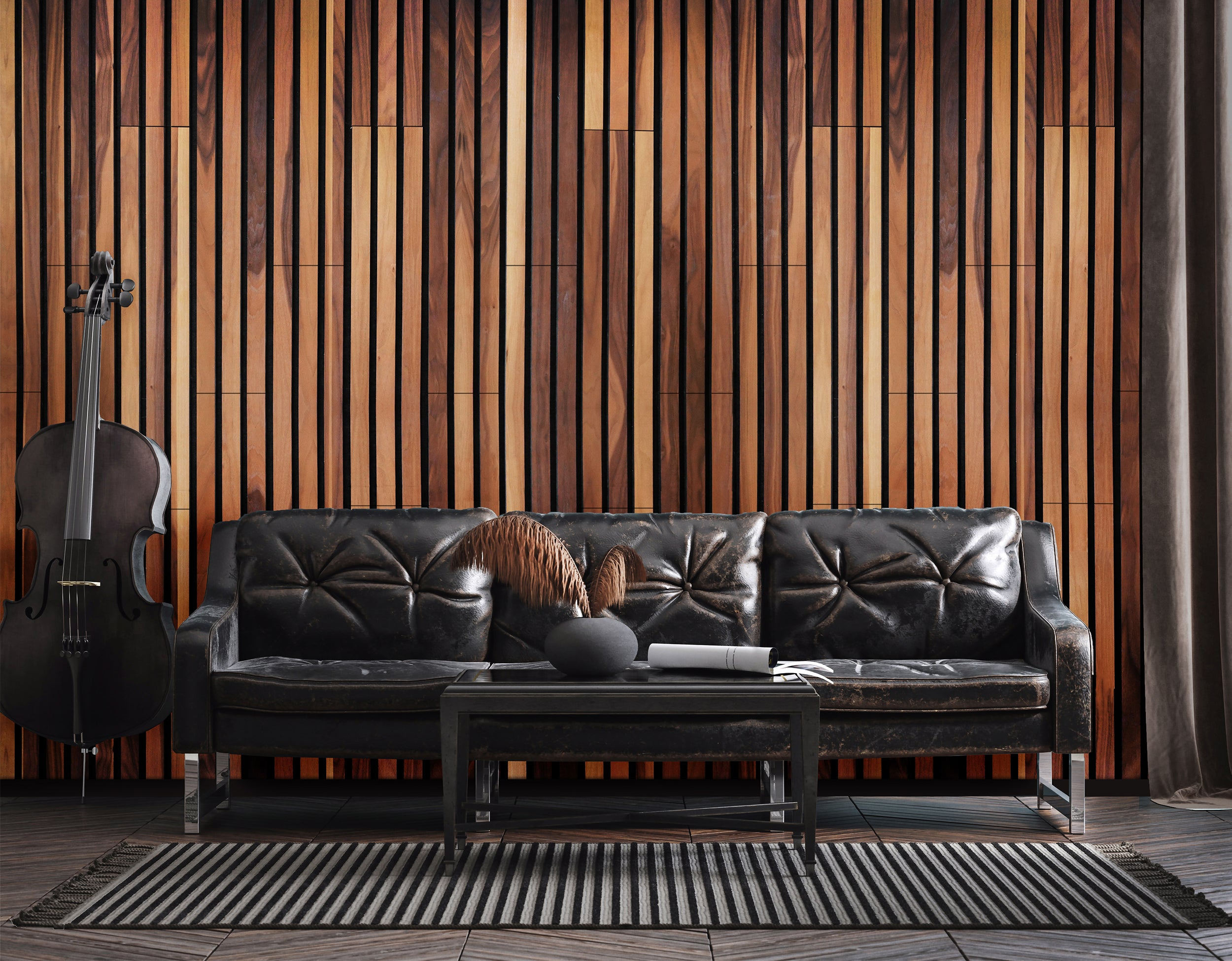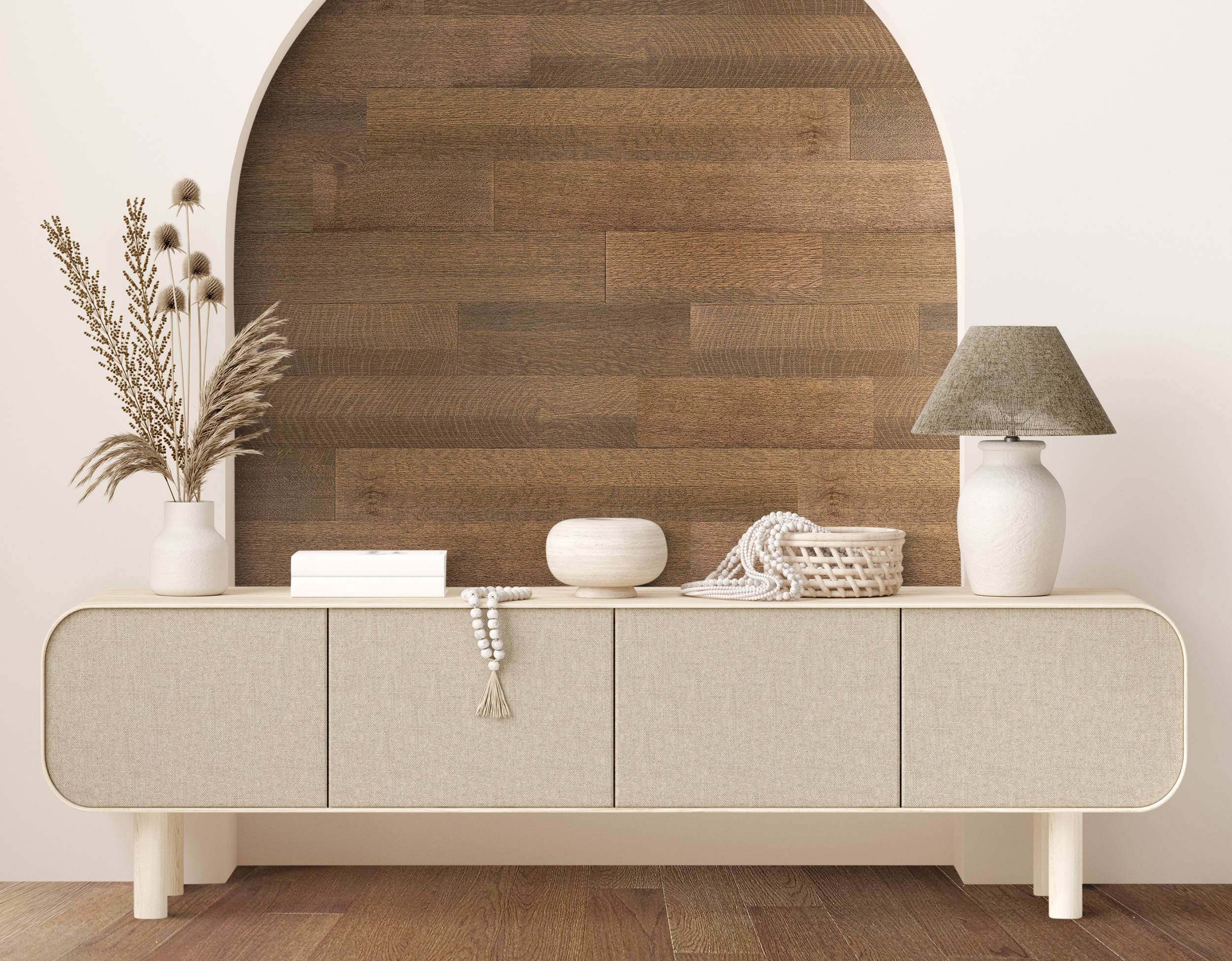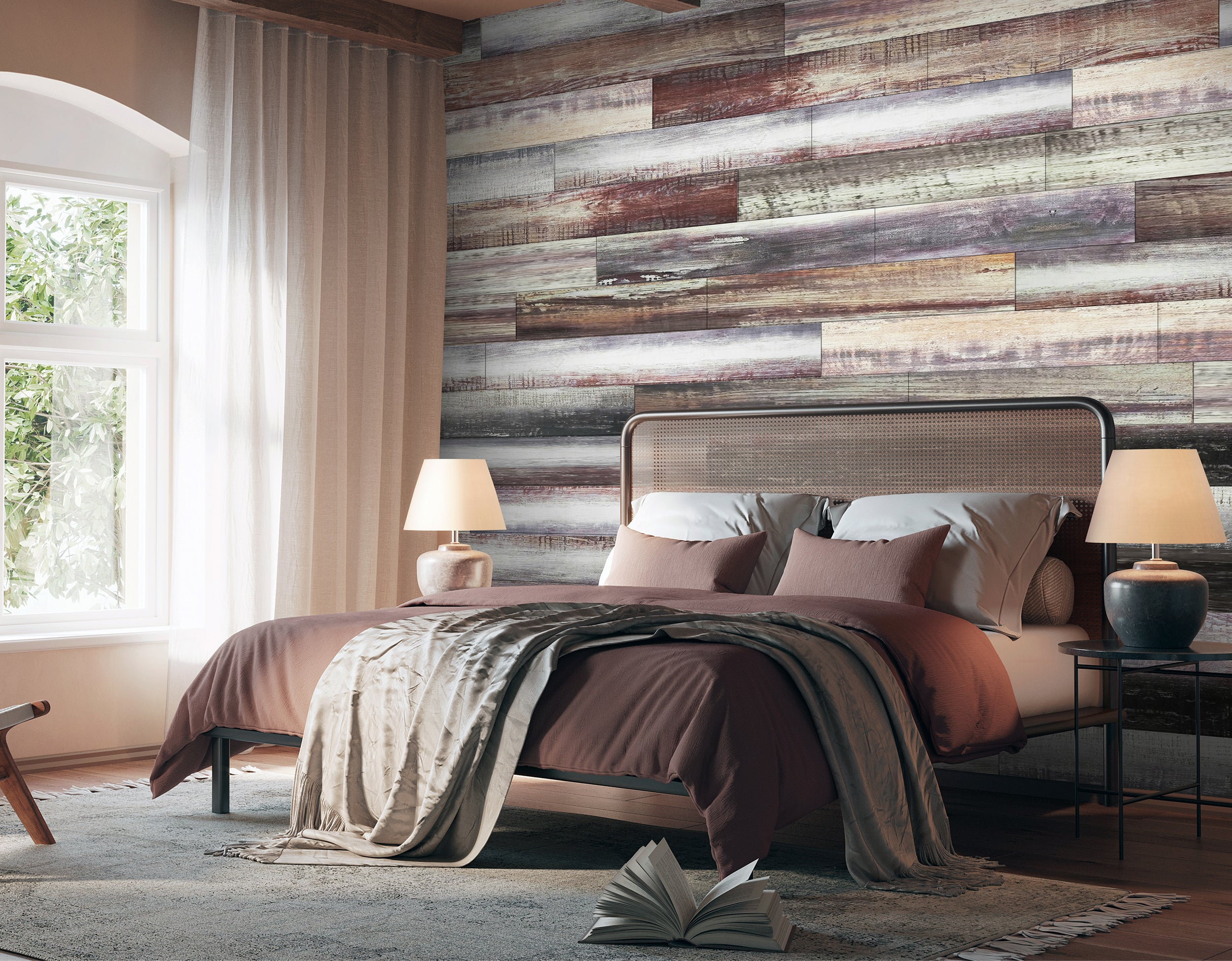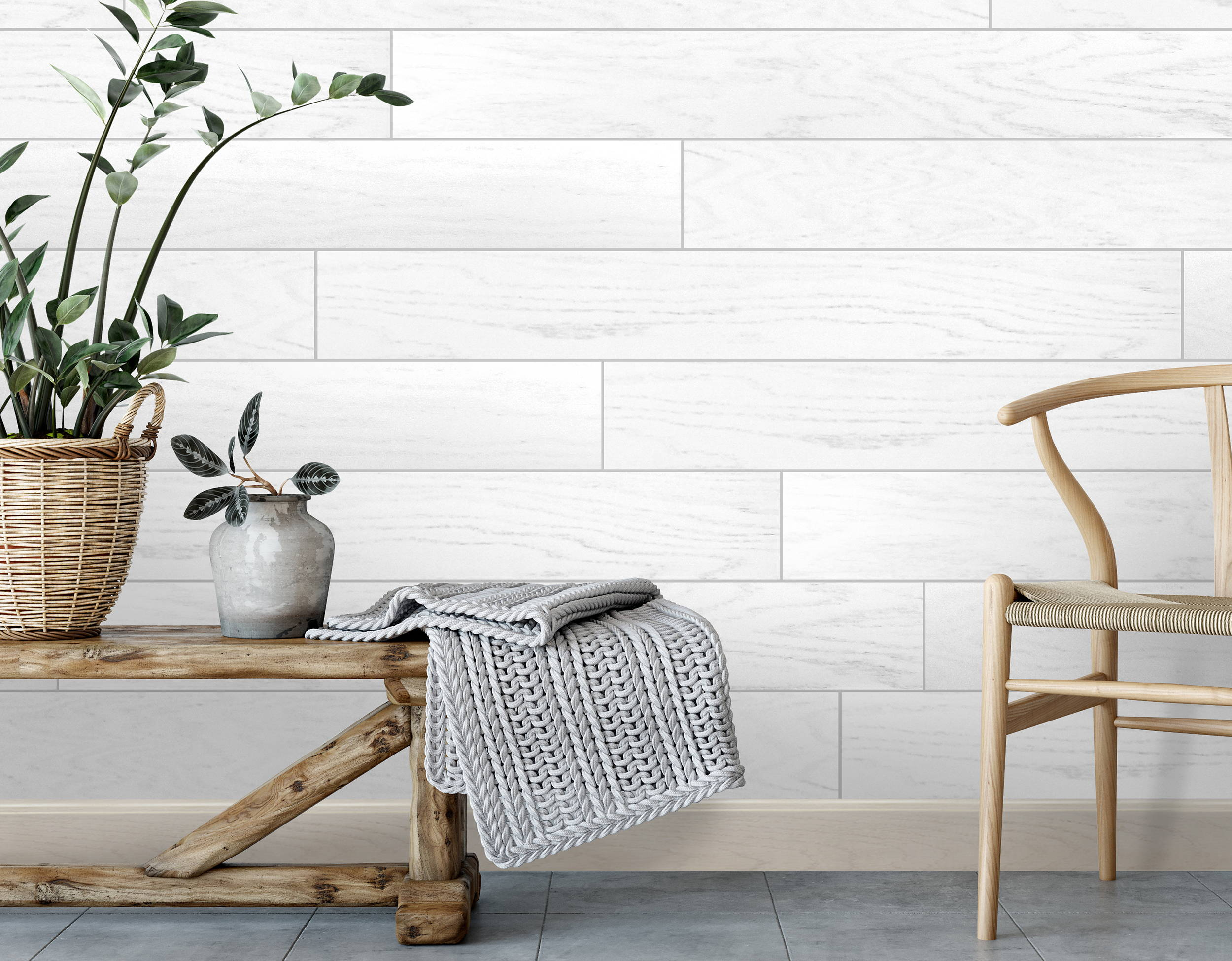### MEASURE & CALCULATE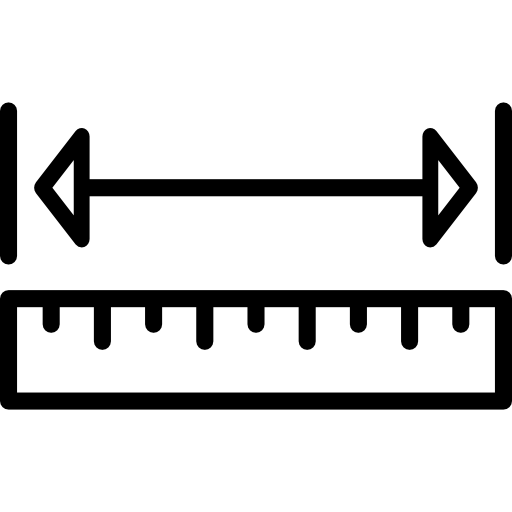### MEASURE & CALCULATE YOUR SURFACES

When measuring your surface, make sure to measure obstructions like doors and windows and to subtract that from your total AREA.

BASE LF x HEIGHT LF = AREA (SQ. FT.)
If calculating the base and the height in inches, divide the total by 144 for total AREA SQ. FT.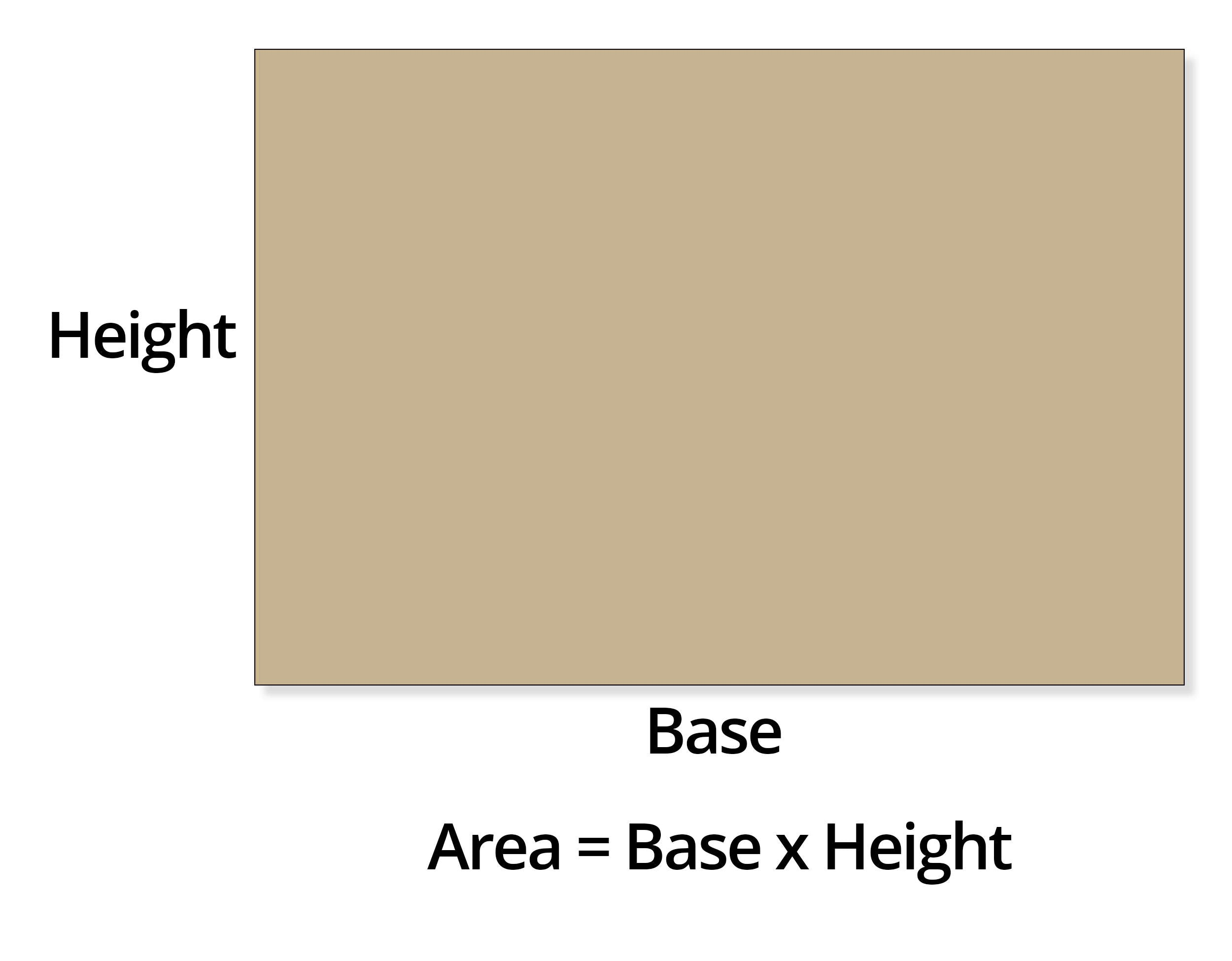When calculating the area of a triangle, you would follow the same rules that you would for a square or a rectangle and divide that total in half.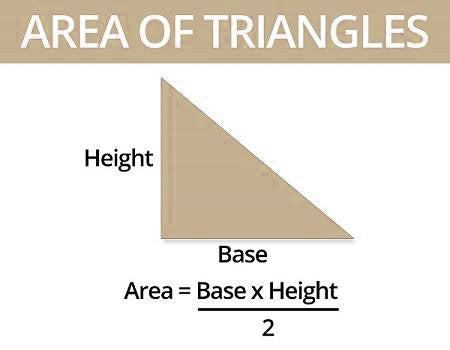### CALCULATE QTY NEEDED & ADD 10%

The 10% extra accounts for waste that occurs during installation.

We make calculating easy for you with our carton calculator tools!